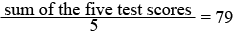SEARCH HOMEMath Central Quandaries & QueriesQuestion from dawn, a parent: After 5 tests, Whitney's average test score was 79. After she scored 85 on the 6th test. What was her new average?Dawn,

When the teacher calculated Whitney's average after 5 tests her calculation wasWhat was the sum of the 5 test scores?

She scored 85 on the 6th test. What was the sum of the 6 test scores?

What was the average of the 6 test scores?

PennyMath Central is supported by the University of Regina and The Pacific Institute for the Mathematical Sciences.